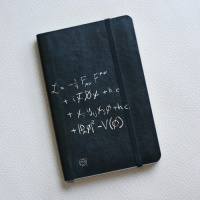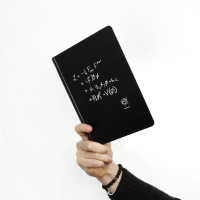# Standard Model formula notebookNotebook with the Langragian equation and the CERN logo.

This equation neatly sums up our current understanding of fundamental particles and forces. It represents mathematically what we call the Standard Model of particle physics. The top line describes the forces: electricity, magnetism and the strong and weak nuclear forces. The second line describes how these forces act on the fundamental particles of matter, namely quarks and leptons. The third line describes how these particles obtain their mass from the Higgs boson, and the fourth line enables the Higgs boson to do the job.

Many experiments at CERN and other laboratories have verified the top two lines in detail. One of the primary objectives of the LHC is to see whether the Higgs boson exists and if it behaves as predicted by the last two lines.

If you want to know more and aren’t afraid of some physics, have a look at a very interesting paper published by three CERN guides.Price
12 CHF
Topic
Standard Model Formula
Category
Stationery
Technical Specifications

Hard cover, elastic band closure, 80 pages, 22 x 14.5 cm.